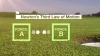# Newton’s Third Law of Motion & Momentum

The impact between a club and the ball produces equal and opposite forces, demonstrating Newton's Third Law of Motion, and also transfers momentum from the club to the ball. "Science of Golf" is produced in partnership with the United States Golf Association and Chevron.

##### transcript

DAN HICKS reporting: Driving, pitching, or putting - golfers know getting just the right amount of force or speed on the ball can be the difference between pulling off the shot...or not.

PATRICK RODGERS (2013 Walker Cup): Golf is such a mental battle out there in the course. And there's definitely a lot that goes into it that you can't really see.

HICKS: Stanford junior Patrick Rodgers is an amateur golfer and a member of the winning 2013 U.S. Walker Cup team.

RODGERS: It really helps to understand how the golf ball and the golf club actually interact and what creates power and speed.

HICKS: When they do interact, it's easy to see that the force of the golf club affects the ball. What's not so obvious is that the golf ball applies that same force toward the club!

JIM HUBBELL (United States Golf Association): During the impact, as the club is applying a force to the ball, the ball is pushing back with an equal and opposite force. And that would be a good illustration of Newton's third law.

HICKS: Newton's Third Law of Motion says that forces act in pairs, equal in size and opposite in direction.

HUBBELL: A force is really any push or pull on an object. It has a direction and it has a magnitude.

HICKS: Force is measured in units called newtons. Say the collision applies 8000 newtons, or 1800 pounds of force, to the ball in this direction. The ball applies the same 8000 newtons of force back to the club in the opposite direction.

HUBBELL: And so during that collision as the moving club contacts that ball, there's going to be interaction of forces between the club and the ball.

HICKS: In a collision, the impulse - the force times how much time the force is applied - will cause the object to speed up or slow down. If the club applies a force of 8000 newtons for 500 microseconds on a ball with a mass of .046 kilograms, then the ball will be moving at 87 meters per second after the collision. The ball applies a force of 8000 newtons back to the club for the same 500 microseconds. But the club has more mass than the ball, so the club's speed doesn't change as much, slowing down only about 13 meters per second. The impulse causes - and is equal to - the change in momentum.

HUBBELL: Momentum is really defined as the mass of an object times its velocity. Momentum is most clearly seen during an impact where the momentum of the club is transferred into momentum in the ball.

HICKS: This transfer of momentum from one object to another is a principle of physics known as the law of Conservation of Momentum, which says that the total momentum before the impact must be equal to the total momentum after the impact. The ball gains momentum because the club pushes on it, and the club loses momentum because the ball pushes back.

HUBBELL: The weight and mass of the club and/or ball will determine how much momentum is transferred to the ball.

HICKS: Before a club and ball collide, each has a certain momentum: the mass of each, multiplied by its velocity. With impact, both have a change in momentum, which is equal to mass multiplied by change in velocity. The ball gains, and the club loses, 4 kilogram meters per second of momentum.

RODGERS: There's so much science that is entailed in the game. And I think to have a complete understanding of the science that is involved in golf not only can help you understand science, but it can better yourself as a golfer.

HICKS: Science that's found in Newton's Third Law of Motion and in the momentum of every swing that gets the ball from the first tee to the last hole.